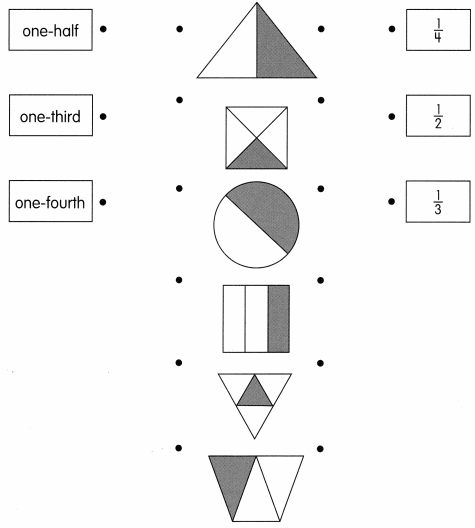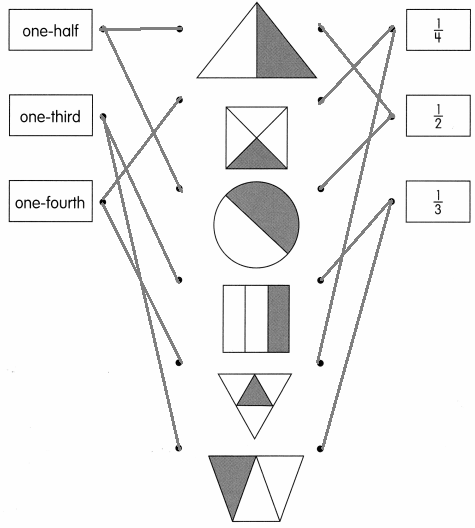# Math in Focus Grade 2 Chapter 12 Practice 1 Answer Key Understanding Fractions

Practice the problems of Math in Focus Grade 2 Workbook Answer Key Chapter 12 Practice 1 Understanding Fractions to score better marks in the exam.

## Math in Focus Grade 2 Chapter 12 Practice 1 Answer Key Understanding Fractions

Put an ✗ in the box if the shape is divided into equal parts.

Example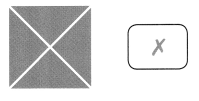Question 1.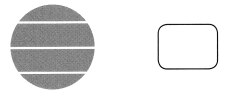The given circle shape is not divided in the equal parts.
There are 4 parts in the circle. These 4 parts are not in the equal shape.

Question 2.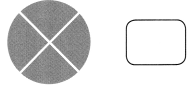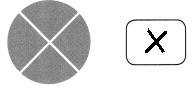The given circle shape is divided into 4 equal parts.
Question 3.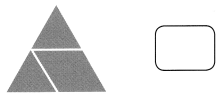The given triangle shape is not divided in the equal parts.
There are 3 parts in the triangle. These 3 parts are not in the equal shape.

Question 4.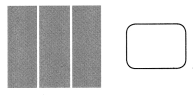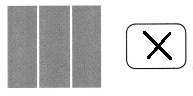The given shape is divided into 3 equal parts.

Question 5.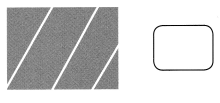The given rectangular shape is not divided in the equal parts.
There are 4 parts in the rectangle. These 4 parts are not in the equal shape.

Question 6.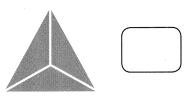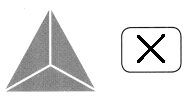The given triangle is divided into 3 equal parts.

Question 7.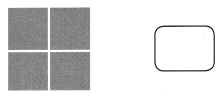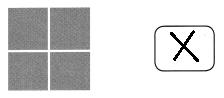The given square is divided into 4 equal parts.

Look at the pictures. Then fill in the blanks.

Example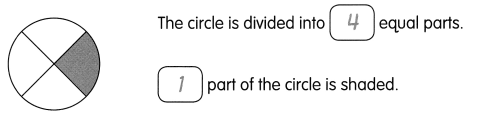Question 8.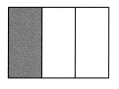The rectangle is divided into ___________ equal parts. ___________ part of the rectangle is shaded.
The rectangle is divided into 3 equal parts. 1 part of the rectangle is shaped.

Circle the fraction and words that match the picture.

Example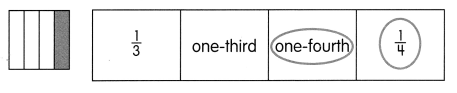Question 9.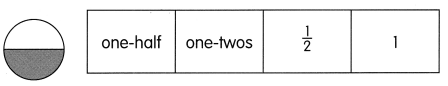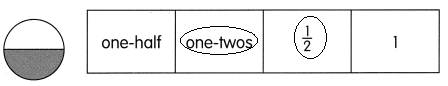The given circle is divided into 2 parts and 1 part is shaded.
Question 10.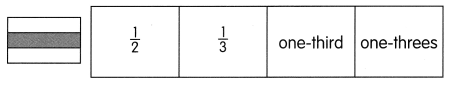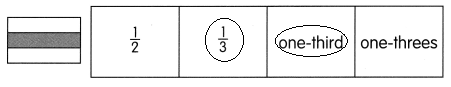The rectangle is divided into 3 parts and 1 part is shaded.

Mark with an ✗ the fractional part that does not belong in each row.

Example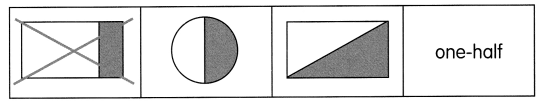Question 11.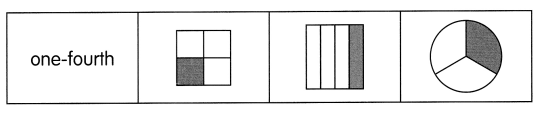In the given figures the wrong image is the circle.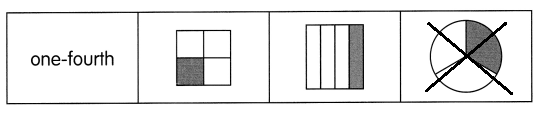Question 12.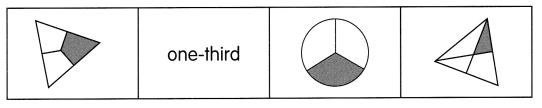In the given figures the wrong image is the triangle that is divided into 4 parts.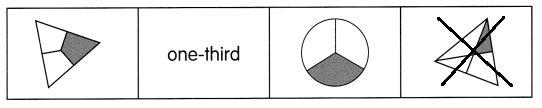Fill in the blanks.

Example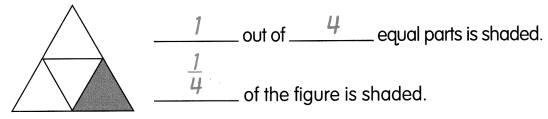Question 13.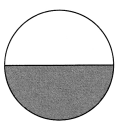__________ out of ___________ equal parts is shaded.
___________ of the figure is shaded.
1 out of 2 equal parts is shaded.
1/2 of the figure is shaded.

Question 14.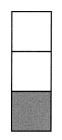___________ out of ___________ equal parts is shaded.
___________ of the figure is shaded.
1 out of 3 equal parts is shaded.
1/3 of the figure is shaded.

Question 15.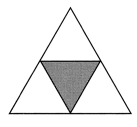___________ out of ___________ equal parts is shaded.
___________ of the figure is shaded.
1 out of 4 equal parts is shaded.
1/4 of the figure is shsded.

Write a fraction for each shaded part.

Example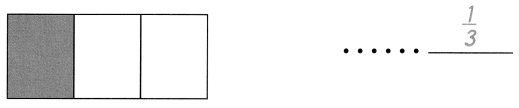Question 16.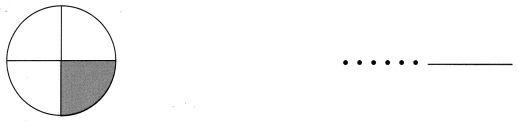The Given circle is divided into 4 parts and 1 part is shaded.
The fraction of the figure is 1/4.

Question 17.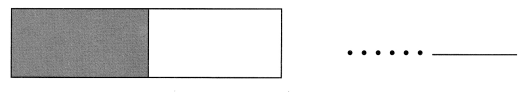The given rectangle is divided into 2 parts and 1 part is shaded.
The fraction of the figure is 1/2.

Question 18.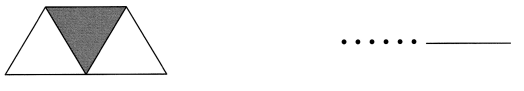The given shape is divided into 3 equal parts and 1 part is shaded.
The fraction of the given figure is 1/3.

Question 19.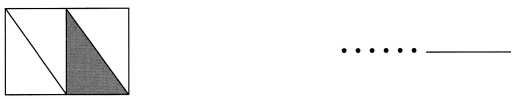The given shape is divided into 4 equal parts and 1 part is shaded.
The fraction of the given figure is 1/4.

Shade part(s) of each figure to show the fraction.

Question 20.
$$\frac{1}{3}$$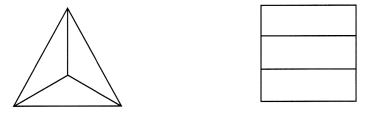$$\frac{1}{3}$$ = 1/3
The given figures is divided into 3 parts. Then shade the 1 part.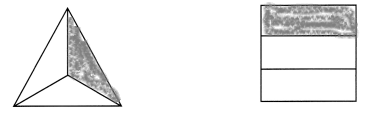Question 21.
$$\frac{1}{4}$$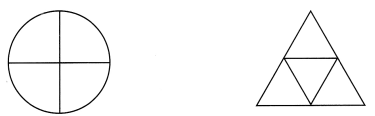$$\frac{1}{4}$$ = 1/4
The given figures is divided into 4 parts. Then shade the 1 part.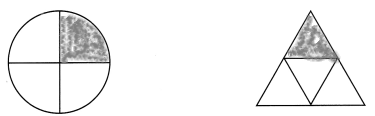Question 22.
$$\frac{1}{2}$$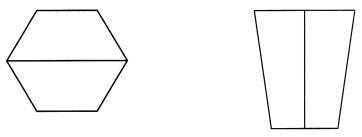$$\frac{1}{2}$$ = 1/2
The given figures are divided into 2 parts. Then shade the 1 part.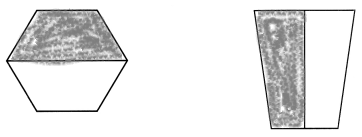Match the words and fractions to the figures.

Question 23.